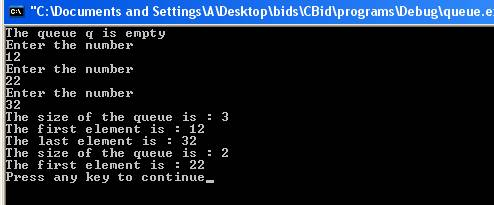Queue

A queue can be automatically created using queue class in C++. A single ended queue is created. The members of the queue are accessed in first in and first out manner.  A header file <queue> is included in the program in order to implement the functionality. The constructor queue() can  create a empty queue. The general form of how variable of type queue is declared is:

queue<type> q;

It creates a queue q of type integer. For example,

queue<int> q;

creates a queue of type integer. Some of the functions of queue are:-

•  Back- The function back() places the reference to the last element in the queue.
•  Empty – The function empty() returns true if queue is empty.
•  Front- The function front() returns a reference to the first element of the queue.
•  Pop- The function pop() removes the first element of the queue.
•  Push – The function push() adds the element at then end of the queue.
•  Size- The function size() returns the size of the queue.

Here is a program which illustrates the working of functions of queue.

#include<iostream>

#include<queue>

using namespace std;

int main ()

{

int i=0,num=0,num1=0;

queue<int> q;

if(q.empty())

{

cout << "The queue q is empty" << endl;

}

for(i=0;i<3;i++)

{

cout << "Enter the number" << endl;

cin >> num;

q.push(num);

}

cout << "The size of the queue is : " << q.size() << endl;

cout << "The first element is : " << q.front() << endl;

cout << "The last element is : " << q.back() << endl;

q.pop();

cout << "The size of the queue is : " << q.size() << endl;

cout << "The first element is : " << q.front() << endl;

return(0);

}

The result of the program is:-The statement

#include<queue>

includes a header file <queue> into the program. The statement

queue<int> q;

creates a queue q of integer type. The statement

if(q.empty())

checks  whether queue is empty or not. It returns true because queue is empty. The statement

q.push(num);

inserts an element num entered by the user at the end of the queue. The statement

cout << "The size of the queue is : " << q.size() << endl;

prints the size of the queue which is 3 after pushing 3 elements in the queue. The statement

cout << "The first element is : " << q.front() << endl;

prints the first element of the queue. The function q.front() returns the first element of the queue. The statement

cout << "The last element is : " << q.back() << endl;

prints the last element of the queue. The function q.back() returns the last element of the queue. The statement

q.pop();

removes the front element of the queue. It reduces the size of the queue by 1. Now the first element will change and second element will become first element and so on.

Go to the previous lesson or proceed to the next lesson